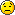Forums

## Extend syntax

General discussion about Cobra. Releases and general news will also be posted here.
Feel free to ask questions or just say "Hello".

### Extend syntax

I'm trying to extend a List of decimal, but getting "Type parameter declaration must be an identifier not a type"

`extend List<of decimal>    def max as decimal        m = decimal.minValue        for i in this            if i > m                m = i        return m    def min as decimal        m = decimal.maxValue        for i in this            if i < m                m = i        return m`

Can someone point me to the right syntax to use for this extension?
torial

Posts: 229
Location: IA

### Re: Extend syntax

Computer says 'no'.

You cant extend a generic instantiation ( even in C#) which is the form you're attempting here
- the error message is referring to the 'of decimal' (decimal recognised as a Type) rather than expected/allowed in generic dcl 'of T' ( T as an identifier)

If you try to make an extension of a generic template which would be the the expected route you hit issues with determining T.minValue/maxValue and inability to do comparisons on generic Types.
C# handles this with placing constraints on the template Type (on classes anyway havent tried on extensions)
but cobra disallows constraints on extensionsi.e
`#Unworkable/wrong in many waysextend List<of T>#   where T must be Number # compile error fm cobra no constraints on extensions    def max as T        m = this # cant use T.minValue as cant determine method existance         for i in this            if i > m   # compile error generics                m = i        return m    def min as T        m = this        for i in this            if i < m # compile error generics                m = i        return mclass X         def main is shared        l = List<of decimal>()        l.add(123.1)        l.add(44.2)        l.add(87.91)        print l.min        print l.max`

You have to do the extension manually
`class DecExtn    """Decimal Extn utility class on a generic instantiation."""    def max(l as List<of decimal>) as decimal is shared        m = decimal.minValue        for i in l            if i > m                m = i        return m            def min(l as List<of decimal>) as decimal is shared        m = decimal.maxValue        for i in l            if i < m                m = i        return m    class X         def main is shared        l = List<of decimal>()        l.add(123.1)        l.add(44.2)        l.add(87.91)        print DecExtn.min(l)        print DecExtn.max(l)`
hopscc

Posts: 632
Location: New Plymouth, Taranaki, New Zealand

### Re: Extend syntax

Thanks hopscc,

I rewrote it so that it wasn't an extension method so I could move on, but was hoping there was a nice/elegant solution. Honestly hadn't tried it in C#, and the only reason I even thought of extending a List of decimals that way was because I was porting a python app that had similar functionality.
torial

Posts: 229
Location: IA

### Re: Extend syntax

A workaround:

Code: Select all
`use System.Linqextend IEnumerable<of T>   def max as T      return Enumerable.max(this)   def min as T      return Enumerable.min(this)class Examples   shared         def main         list = [0.1d, 0.2d, 0.3d]         min = list.min         max = list.max         print "min:[min], max:[max] -- [list] -- [list.getType]"         print         .more      def more         .minmax<of decimal>( [-0.3d, -0.2d, -0.1d] )         .minmax<of float>( [1.1f, 1.2f, 1.3f] )         .minmax<of int>( [1,2,3] )         .minmax<of int>( @[10, 11, 12])         .minmax<of int>( {65:"A", 66:"b", 67:"c"}.keys )         list = List<of int?>()         list.add(nil)         list.add(1)         list.add(2)         .minmax<of int?>(list)      def minmax<of T>(sequence as IEnumerable<of T>)         min = sequence.min         max = sequence.max         print "min:[min], max:[max] -- [sequence] -- [sequence.getType]"`
thriwkin

Posts: 26